Courses

# RD Sharma Solutions (Part - 2) - Ex-23.1, Data Handling II Central Values, Class 7, Math Class 7 Notes | EduRev

## Class 7: RD Sharma Solutions (Part - 2) - Ex-23.1, Data Handling II Central Values, Class 7, Math Class 7 Notes | EduRev

The document RD Sharma Solutions (Part - 2) - Ex-23.1, Data Handling II Central Values, Class 7, Math Class 7 Notes | EduRev is a part of the Class 7 Course RD Sharma Solutions for Class 7 Mathematics.
All you need of Class 7 at this link: Class 7

#### Question 14:

The percentage of marks obtained by students of a class in mathematics are:
64, 36, 47, 23, 0, 19, 81, 93, 72, 35, 3, 1. Find their mean.

We have :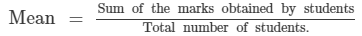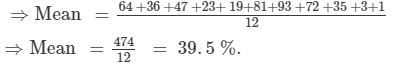#### Question 15:

The numbers of children in 10 families of a locality are:
2, 4, 3, 4, 2, 3, 5, 1, 1, 5. Find the mean number of children per family.

The mean number of children per family =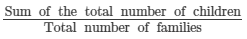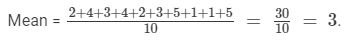Thus, on an average there are 3 children per family in the locality.

#### Question 16:

The mean of marks scored by 100 students was found to be 40. Later on it was discovered that a score of 53 was misread as 83. Find the correct mean.

We have:

n = The number of observations = 100,  Mean = 40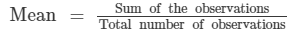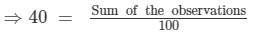⇒ 40 ×100 = Sum of the observations

Thus, the incorrect sum of the observations = 40 x 100 = 4000.

Now,

The correct sum of the observations = Incorrect sum of the observations - Incorrect observation + Correct observation

⇒ The correct sum of the observations = 4000 - 83 + 53
⇒ The correct sum of the observations = 4000 - 30 =  3970

∴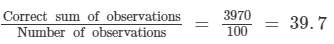#### Question 17:

The mean of five numbers is 27. If one number is excluded, their mean is 25. Find the excluded number.

We have: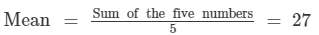So, sum of the five numbers = 5 ×27 = 135.

Now,

The mean of four numbers =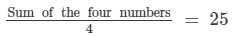So, sum of the four numbers = 4 ×25  = 100.

Therefore, the excluded number  = Sum of the five numbers - sum of the four numbers

⇒ The excluded number = 135 - 100 = 35.

#### Question 18:

The mean weight per student in a group of 7 students is 55 kg. The individual weights of 6 of them (in kg) are 52, 54, 55, 53, 56 and 54. Find the weight of the seventh student.

We have: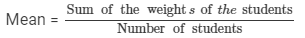Let the weight of the seventh student be x kg.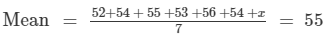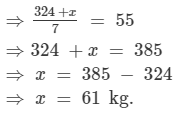Thus, the weight of the seventh student is 61 kg.

#### Question 19:

The mean weight of 8 numbers is 15 kg. If each number is multiplied by 2, what will be the new mean?

Let x1, x2x3...x8 be the eight numbers whose mean is 15 kg. Then,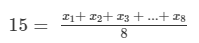x1, x2x3...x8  = 15 x 8

⇒ x1, x2x3...x8 = 120

Let the new numbers be 2x1 , 2x2, 2x3, ...2x8. Let M be the arithmetic mean of the new numbers.
Then,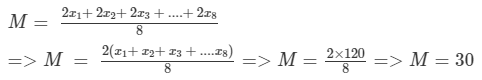#### Question 20:

The mean of 5 numbers is 18. If one number is excluded, their mean is 16. Find the excluded number.

Let x1,x2,x3,x4 & x5x1,x2,x3,x4 & x5 be five numbers whose mean is 18. Then,
18 = Sum of five numbers ÷ 5
∴ Sum of five numbers = 18 × 5 = 90.

Now, if one number is excluded, then their mean is 16.
So,
16= Sum of four numbers ÷ 4
∴ Sum of four numbers = 16 × 4 = 64.

The excluded number =  Sum of the five observations - Sum of the four observations

∴ The excluded number = 90 - 64
∴ The excluded number = 26.

#### Question 21:

The mean of 200 items was 50. Later on, it was discovered that the two items were misread as 92 and 8 instead of 192 and 88. Find the correct mean.

n = Number of observations = 200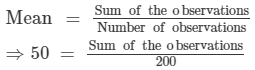⇒Sum of the observations = 50 × 200 = 10,000

Thus, the incorrect sum of the observations = 50 x 200
Now,
The correct sum of the observations = Incorrect sum of the observations - Incorrect observations + Correct observations
⇒Correct sum of the observations =  10,000 - (92+ 8) + (192 + 88)

⇒ Correct sum of the observations = 10,000 - 100 + 280
⇒ Correct sum of the observations = 9900 +280
⇒ Correct sum of the observations = 10180.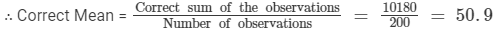#### Question 22:

The mean of 5 numbers is 27. If one more number is included, then the mean is 25. Find the included number.

We have:
Mean =  Sum of  five numbers ÷ 5
⇒ Sum of the five numbers = 27 x 5 = 135.

Now, New mean = 25
25 = Sum of six numbers ÷÷ 6
⇒ Sum of the six numbers = 25 x 6 = 150.

The included number = Sum of the six numbers - Sum of the five numbers
⇒The included number = 150 - 135
⇒The included number =  15.

#### Question 23:

The mean of 75 numbers is 35. If each number is multiplied by 4, find the new mean.

Let x1, x2, x3, ... x75 be 75 numbers with their mean equal to 35. Then,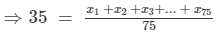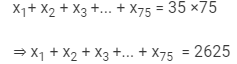The new numbers are 4x1, 4x2, 4x3, ...4x75. Let M be the arithmetic mean of the new numbers. Then,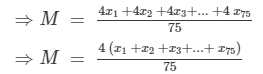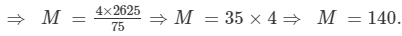The document RD Sharma Solutions (Part - 2) - Ex-23.1, Data Handling II Central Values, Class 7, Math Class 7 Notes | EduRev is a part of the Class 7 Course RD Sharma Solutions for Class 7 Mathematics.
All you need of Class 7 at this link: Class 7Use Code STAYHOME200 and get INR 200 additional OFF Use Coupon Code
All Tests, Videos & Notes of Class 7: Class 7

### Top Courses for Class 7## RD Sharma Solutions for Class 7 Mathematics

97 docs

### Top Courses for Class 7Track your progress, build streaks, highlight & save important lessons and more!

,

,

,

,

,

,

,

,

,

,

,

,

,

,

,

,

,

,

,

,

,

,

,

,

,

,

,

,

,

,

;